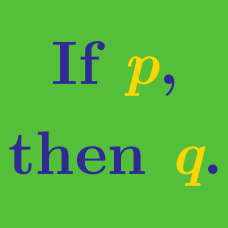Logic

# Operator Search Warmup

What sign, in place of $$\square$$, will make the equation true?

$\large 6 \square 2 = 4$

Using only $$+$$ and $$-$$, can we fill in the boxes to make the following expression true:

$\large 6 \square 4 \square 2 = 8 ?$

Out of the 4 standard arithmetic operations ($$+, - , \times, \div$$), can we place them into $$\square$$ such that the equation is true?

$\large 1 \square 2 \square 3 = 6$

Note: Each $$\square$$ could use the same or a different operation.

Using only $$+$$ and $$-$$, can we fill in the boxes to make the following expression true:

$\large 1 \square 2 \square 3 \square 4 \square 5 = 10 ?$

Out of the 4 signs ($$+, -, \times, \div$$), how many of them could be placed in $$\square$$ to make the statement true?

$\large 2 \square 2 = 4$

×

Problem Loading...

Note Loading...

Set Loading...